## Electric Circuits Review

### Part A: Multiple-Multiple Choice

1. Which of the following statements are true about an electric circuit? List all that apply.

1. Electrons are the mobile charge carriers in an electric circuit.
2. The path of charge flow from the + to the - terminal of the circuit can consist of nonconductive material.
3. In an electric circuit of an automobile, the 12-Volt car battery is sometimes referred to as the internal circuit because it is located inside of the hood of the car.
4. Charge is supplied with energy in the internal circuit and the energy is transformed into other forms in the external circuit.
5. Charge is consumed as it moves through the energy-transforming devices of the external circuit. For instance, the amount of charge entering a light bulb in a second is less than that which exits the light bulb in a second.
6. A battery is used to power a flashlight circuit. When the battery no longer works, it is because it has run out of charge.
7. The location on an electric circuit where the charge possesses the greatest amount of electric potential energy is the + terminal of the battery.

2. Which of the following statements are true about an electric potential or electric potential difference? List all that apply.

1. Electric potential can be expressed in units of volts.
2. Electric potential can also be expressed in units of Joules.
3. The electric potential of a charge at a given location provides a measure of the rate at which charge flows past that point.
4. Work must be done on a + charge to move it against (i.e., in the opposite direction of) an electric field.
5. As a + charge moves in the same direction as an electric field, it gains electric potential.
6. The electric potential difference between two points is simply the difference in potential energy possessed by charge between those two points.
7. If a unit of charge has a high electric potential at any given location, then that charge possesses a large amount of electric potential energy at that location.
8. The + terminal of a battery is a location with a higher electric potential than the - terminal of a battery.
9. Charge flowing through a battery will experience a gain in electric potential.
10. A 6-Volt battery would provide 12 Joules of energy to 2 Coulombs of charge as it is moved from the - to the + terminal.

3. Which of the following statements are true about electric current? List all that apply.

1. Electric current is measured in units of Amperes.
2. Electric current and drift speed are synonymous terms.
3. Electric current is defined as the number of Coulombs of charge which move past a point on a circuit.
4. Electric current is equal to the number of Coulombs of charge which move past a point on a circuit per unit of time.
5. Electric current provides a measure of how fast charge moves between two points on a circuit.
6. The electric current diminishes in value as charge progresses to locations further and further from the + terminal of the battery.
7. The electric current in a circuit will increase as the electric potential impressed across a circuit is increased.
8. The electric current in a circuit will triple in value as the electric potential impressed across a circuit is increased by a factor of three.
9. Suppose a miniature light bulb is connected to a battery in a circuit. A light bulb with a greater resistance will have a greater current.
10. Wider conducting wires are capable of carrying larger currents.
11. If one starts getting technical about the meaning of electric current, one could argue that electric current is not something that flows past a point on a circuit, but rather something that exists to a given measure at a point on a circuit.

4. Which of the following statements are true about an electric resistance? List all that apply.

1. The resistance of an electric circuit is a measure of the overall amount of hindrance to the flow of charge through the circuit.
2. A large resistance value indicates that the charge is encountering a relatively large amount of difficulty in moving through the circuit.
3. The unit of resistance is the ampere.
4. A 5-ohm resistor would allow a current of 0.5 Amperes when 2.5 Volts is impressed across it.
5. A 10-ohm resistor would allow a current of 2 Amperes when 5 Volts is impressed across it.
6. The resistance of a conducting wire will increase as the length of the wire is increased.
7. The resistance of a conducting wire will increase as the cross-sectional area of the wire is increased.
8. Increasing the resistance of an electric circuit will cause the current in the circuit to increase.
9. A threefold increase in the resistance of an electric circuit will result in a threefold decrease in the electric current.
10. A miniature light bulb with a specific resistance is connected to a 1.5-Volt battery to form a circuit. If it were connected to a 6-Volt battery instead, its resistance would increase by a factor of 4.

5. Which of the following statements are true about electric power and electric potential energy? List all that apply.

1. Power is the amount of energy used by an electrical appliance.
2. Power is the rate at which energy is used by an electric circuit.
3. A kiloWatt•hour is a unit of electric power.
4. A Joule/second is a unit of power.
5. A 60-Watt light bulb would consume 30 Joules of electrical energy in 2 seconds.
6. A 120-Watt light bulb would consume 1200 Joules of electrical energy in 6 seconds.
7. A charge moving through an electric circuit would gain electric potential energy in the internal circuit and lose electric potential energy in the external circuit.
8. Charge has the greatest amount of electric potential energy at the - terminal of the battery.
9. If a 60-Watt light bulb is placed in a 120-Volt circuit, then the current in the light bulb is 2 Amps.
10. Consider two light bulbs with different power ratings. A 60-Watt light bulb has a greater resistance than a 100-Watt light bulb.

6. Which of the following statements are true about a series circuit? List all that apply.

1. Series circuits are characterized by the fact that there is a single pathway by which charge can travel from the + terminal to the - terminal.
2. During any single loop of charge around a series circuit, the charge will pass through each resistor in the circuit.
3. The overall resistance of a circuit increases as more and more resistors are placed in series in the circuit.
4. The total current in a circuit increases as more and more resistors are placed in series in the circuit.
5. Suppose that three identical resistors with a resistance of 3-ohms are placed in series with a 12-Volt battery. The current in the circuit is 4 Amps.
6. Suppose that three identical resistors with a resistance of 3-ohms are placed in series with a 12-Volt battery. The electric potential difference across each resistor is 12 Volts.
7. Suppose that two identical resistors are placed in series with a 12-Volt battery. The current in the circuit is 6 amps. The resistance of each resistor is 4 ohms.

7. Which of the following statements are true about a parallel circuit? List all that apply.

1. Parallel circuits are characterized by the fact that there are multiple pathways by which charge can travel from the + terminal to the - terminal.
2. During any single loop of charge around a parallel circuit, the charge will pass through each resistor in the circuit.
3. The overall resistance of a circuit increases as more and more resistors are placed in parallel in the circuit.
4. The total current in a circuit increases as more and more resistors are placed in parallel in the circuit.
5. Suppose that three identical resistors with a resistance of 3-ohms are placed in parallel with a 12-Volt battery. The current in the circuit is 1 Amp.
6. Suppose that three identical resistors with a resistance of 3-ohms are placed in parallel with a 12-Volt battery. The electric potential difference across each resistor is 12 Volts.
7. Suppose that two identical resistors are placed in parallel with a 12-Volt battery. The overall current in the circuit is 6 amps. The resistance of each resistor is 4 ohms.

### Part B: Multiple Choice

8. If an electric circuit was analogous to a water park, then the battery would be analogous to the ____.

a. pipes which carry the water through the water circuit

b. pump which supplies energy to move the water from the ground to a high elevation

c. the people which flow from the top of the water ride to the bottom of the water ride

d. the rate at which water is pumped onto the slide

e. the change in potential energy of the riders

f. the top of the water slide

g. the bottom of the water slide

h. the long lines which exist at the park

i. the speed at which riders move as they slide from the top to the bottom of the ride

9. If an electric circuit was analogous to a water park, then the positive terminal of the battery would be analogous to the ____.

a. pipes which carry the water through the water circuit

b. pump which supplies energy to move the water from the ground to a high elevation

c. the people which flow from the top of the water ride to the bottom of the water ride

d. the rate at which water is pumped onto the slide

e. the change in potential energy of the riders

f. the top of the water slide

g. the bottom of the water slide

h. the long lines which exist at the park

i. the speed at which riders move as they slide from the top to the bottom of the ride

10. If an electric circuit was analogous to a water park, then the electric current would be analogous to the ____.

a. pipes which carry the water through the water circuit

b. pump which supplies energy to move the water from the ground to a high elevation

c. the people which flow from the top of the water ride to the bottom of the water ride

d. the rate at which water is pumped onto the slide

e. the change in potential energy of the riders

f. the top of the water slide

g. the bottom of the water slide

h. the long lines which exist at the park

i. the speed at which riders move as they slide from the top to the bottom of the ride

11. The potential energy possessed per unit of charge at any given location is referred to as the electric ___.

 a. current b. resistance c. potential d. power

12. One ampere is the amount of current that exists when ____ flows by a certain point in a conductor in ____.

 a. one watt; one second b. one joule; one hour c. one electron; one second d. one electron; one hour e. one volt; one second f. one volt; one hour g. one coulomb; one second h. one coulomb; one hour

13. If 6 coulombs of charge flow past point 'A' in a circuit in 4 seconds, then ____ coulombs of charge will flow past point 'A' in 8 seconds.

 a. 0.67 b. 1.5 c. 2 d. 3 e. 4 f. 6 g. 8 h. 12 i. 24

14. In which of the following situations will the light bulb light? List all that apply.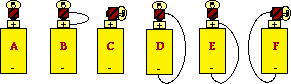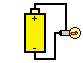For Questions #15-#17:

A simple circuit containing a battery and a light bulb is shown in the diagram at the right. Use this diagram to answer the next several questions.

15. The current through the battery is ___.

 a. greater than that through the light bulb b. less than that through the light bulb c. the same as that through the light bulb d. greater than that through each wire e. less than that through each wire

16. Charge flowing through this circuit is most energized at ____. Choose the one best answer.

a. the + terminal of the battery

b. the - terminal of the battery

c. just prior to entering the light bulb

d. just after exiting the light bulb

e. ... nonsense! The energy of the charge is the same everywhere throughout the circuit.

17. The role or purpose of the battery in this circuit is to ____. Choose three.

a. supply electric charge so that a current can exist

b. supply energy to the charge

c. move the charge from the - to the + terminal of the battery

d. transform energy from electrical energy into light energy

e. establish an electric potential difference between the + and - terminals

f. replenish the charge which is lost in the light bulb

g. offer resistance to the flow of charge so that the light bulb can get hot

18. A 12-Volt battery would supply ___. List all that apply.

a. 3 Coulombs of charge with 4 Joules of energy

b. 4 Coulombs of charge with 3 Joules of energy

c. 12 Coulombs of charge with 1 Joule of energy

d. 1 Coulomb of charge with 12 Joules of energy

e. 0.5 Coulombs of charge with 24 Joules of energy

f. 24 Coulombs of charge with 2 Joules of energy

19. The charges that flow through the wires in your home ____.

a. are stored in the outlets at your home

b. are created when an appliance is turned on

c. originate at the power (energy) company

d. originate in the wires between your home and the power company

20. Approximately how long would it take an electron to travel from the battery of a car to a head light and back (complete loop)?

 a. seconds b. hours c. years d. one-millionth of a second e. one-tenth of a second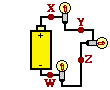21. The electric circuit shown at the right consists of a battery and three identical light bulbs. Which of the following statements are true concerning this circuit? List all that apply.

a. The current through point X will be greater than that through point Z.

b. The current through point Z will be greater than that through point Y.

c. The current will be the same through points X, Y and Z.

d. The current through point X will be greater than that through point Y.

e. The current through point Y will be greater than that through point X.

22. The electric circuit shown at the right consists of a battery and three identical light bulbs. Which of the following statements are true concerning this circuit? List all that apply.

a. The electric potential difference between X and Y is more than that between Y and Z.

b. The electric potential difference between X and Z is more than that between Y and W.

c. The electric potential difference between X and Y is the same as that between Y and Z.

d. The electric potential difference between X and Z is the same as that between Y and W.

e. The electric potential difference between Y and W is more than that between X and Y.23. The electric circuit shown at the right consists of a battery and three identical light bulbs. Which of the following statements are true concerning this circuit? List all that apply.

a. Conventional current is directed through the external circuit from point X to Y to Z to W.

b. Conventional current is directed through the external circuit from point W to Z to Y to X.

c. Conventional current is directed through the internal circuit from point W to point X.

d. Conventional current is directed through the internal circuit from point X to point W.

e. The point where charge possesses the least amount of electric potential energy is point W.

24. Voltage ____ an electrical circuit.

 a. goes through b. is expressed across c. is constant throughout d. is the rate at which charges move through

25. Two or more of the following words and phrases mean the same thing. Identify them by listing their letters.

 a. Voltage b. Wattage c. Electric Potential Difference d. Rate at which charge flows e. Electric Pressure f. Energy

26. A high voltage battery can ____.

a. do a lot of work on each charge it encounters

b. do a lot of work over the course of its lifetime

c. push a lot of charge through a circuit

d. last a long time

27. Which one of the following occurs when a rechargeable battery is recharged?

a. The battery, which has run out of watts, has its wattage restored.

b. The battery, which has run out of amps, has current placed back into it.

c. The battery, which has run out of charge, has charge returned to it.

d. The battery, which has run out of chemical reactants, has its chemicals reformed.

28. Birds can safely stand on high voltage electric power lines. This is because ____.

a. they are at low potential with respect to the ground.

b. they offer no resistance to current.

c. they always choose power lines that are not in use.

d. the potential difference between their feet is low.

e. they are perfect insulators.

f. they are perfect conductors.

29. When the light bulb in your lamp no longer works, it is because the bulb has _____.

a. run out of energy and can no longer pump charge

b. run out of voltage and must be recharged

c. run out of electrons and so there is no more current

d. burned all of its watts and can no longer shine

e. tripped a circuit breaker and must be fixed at the fuse box

f. a broken filament which has resulted in an open circuit

g. ... nonsense! The bulb is fine; your family just needs to fully pay their power bill.

30. A battery is needed in the circuit of your flashlight so that ____.

a. charge is provided to the wires

b. the energy of the light is balanced by the battery

c. an exothermic, light-creating reaction is possible

d. an electric potential difference is maintained across the circuit

e. electrons are supplied in order to light the bulb

31. When you turn on the room lights, they light immediately. This is best explained by the fact that ____.

a. electrons move very fast from the switch to the light bulb filament

b. electrons present everywhere in the circuit move instantly

32. The drift velocity of mobile charge carriers in electric circuits is ____.

a. very fast; less than but very close to the speed of light

b. fast; faster than the fastest car but nowhere near the speed of light

c. slow; slower than Michael Jackson runs the 220-meters

d. very slow; slower than a snail

33. Suppose that the current in a typical circuit (DC) is large. This is an indication that ____.

a. the mobile charge carriers are moving very fast

b. a large number of mobile charge carriers are moving forward per second

c. both a and b are true

34. Which of the following statements represent correct unit equivalencies? List all that apply.

 a. 1 Ampere = 1 Coulomb / second b. 1 Joule = 1 Volt / Coulomb c. 1 Watt = 1 Joule • second d. 1 Watt = 1 Volt • Coulomb / second e. 1 Joule / Ohm = 1 Ampere • Coulomb f. 1 Joule • Ohm = 1 Volt2 • second

35. Which of the following diagrams represents resistors connected in in series? List all that apply.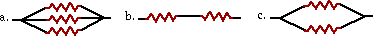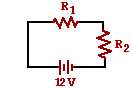Questions #36-#39:

The diagram at the right shows two identical resistors - R1 and R2 - placed in a circuit with a 12-Volt battery. Use this diagram to answer the next several questions.

36. These two resistors are connected in ____.

 a. series b. parallel c. neither

37. The electric potential difference (voltage drop) across each resistor is ___ Volts.

 a. 6 b. 12 c. 24 d. ... nonsense!. The electric potential difference is dependent upon the actual resistance of the resistors

38. If a third resistor (R3), identical to the other two, is added in series with the first two, then the overall resistance will ____ and the overall current will ____.

 a. increase, increase b. decrease, decrease c. increase, decrease d. decrease, increase e. increase, remain the same f. decrease, remain the same g. remain the same, increase h. remain the same, decrease i. remain the same, remain the same

39. If a third resistor (R3), identical to the other two, is added in series with the first two, then the electric potential difference (voltage drop) across each of the three individual resistors will ____.

 a. increase b. decrease c. remain the sameQuestions #40-#43:

The diagram at the right shows two identical resistors - R1 and R2 - placed in a circuit with a 12-Volt battery. Use this diagram to answer the next several questions.

40. These two resistors are connected in ____.

 a. series b. parallel c. neither

41. The electric potential difference (voltage drop) across each resistor is ___ Volts.

 a. 6 b. 12 c. 24 d. ... nonsense!. The electric potential difference is dependent upon the actual resistance of the resistors

42. If a third resistor (R3), identical to the other two, is added in parallel with the first two, then the overall resistance will ____ and the overall current will ____.

 a. increase, increase b. decrease, decrease c. increase, decrease d. decrease, increase e. increase, remain the same f. decrease, remain the same g. remain the same, increase h. remain the same, decrease i. remain the same, remain the same

43. If a third resistor (R3), identical to the other two, is added in parallel with the first two, then the electric potential difference (voltage drop) across each of the three individual resistors will ____.

 a. increase b. decrease c. remain the same

44. The resistance of a charge-carrying conducting wire will increase as the ____. Choose all that apply.

a. length of the wire is increased

b. cross-sectional area of the wire is increased

c. temperature of the wire is increased

d. voltage impressed across the ends of the wire is increased

e. wire is placed closer and closer to the + terminal of the circuit

45.   When plugged into a 120-Volt outlet, a light bulb consumes 300 joules of energy over a 5 second time period. The power of the light bulb is ____ Watts.

 a. 0.0167 b. 0.50 c. 2.0 d. 2.50 e. 60 f. 600 g. 1500 h. 7200

46. A certain electrical circuit contains a battery, wires and a light bulb. If potential energy is gained by charges at the battery location, then charges lose potential energy ____.

a. in the wires only

b. in the bulb only

c. equally in the wires and the bulb

d. mostly in the wires but a little in the bulb

e. mostly in the bulb but a little in the wires

f. nowhere

47. A high resistance light bulb and a low resistance light bulb are connected in series to a 6-Volt pack of batteries. Which of the two light bulbs will shine the brightest?

a. They will have the same brightness.

b. The low-R bulb will shine more brightly.

c. The high-R bulb will shine more brightly.

d. There is no way to make such a prediction since bulb brightness is independent of bulb resistance.

48. A high resistance light bulb and a low resistance light bulb are connected in parallel and powered by a 6-Volt pack of batteries. Which of the two light bulbs will shine the brightest?

a. They will have the same brightness.

b. The low-R bulb will shine more brightly.

c. The high-R bulb will shine more brightly.

d. There is no way to make such a prediction since bulb brightness is indepenent of bulb resistance.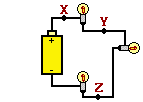49. Three identical light bulbs are connected to a battery as shown at the right. Which adjustments could be made to the circuit that would increase the current being measured at X? Include all that apply.

a. increase the resistance of one of the bulbs

b. increase the resistance of two of the bulbs

c. decrease the resistance of two of the bulbs

d. increase the voltage of the battery

e. decrease the voltage of the battery

f. remove one of the bulbs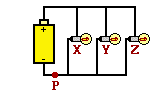50. Three identical light bulbs (labeled X, Y and Z) are connected to a battery as shown at the right. Which adjustments could be made to the circuit below that would increase the current at point P? List all that apply.

a. increase the resistance of one of the bulbs

b. increase the resistance of two of the bulbs

c. decrease the resistance of two of the bulbs

d. increase the voltage of the battery

e. decrease the voltage of the battery

f. remove one of the bulbs

51. Three identical light bulbs (labeled X, Y and Z) are connected to a battery as shown at the right. Which adjustments could be made to the circuit below that would decrease the current in bulb Z? List all that apply.

a. increase the resistance of bulb X

b. decrease the resistance of bulb X

c. increase the resistance of bulb Z

d. decrease the resistance of bulb Z

e. increase the voltage of the battery

f. decrease the voltage of the battery

g. remove bulb Y

### Part C: Diagramming and Analysis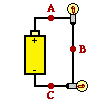52. Consider the diagram at the right to answer the following questions.

a. If 4 Coulombs of charge flow past point A in 2 seconds, then ___ Coulombs of charge flow past point B in 2 seconds.

 a. less than 4 b. 4 c. more than 4 d. impossible to make such a prediction without knowledge of the resistances.

b. If 4 Coulombs of charge flow past point A in 2 seconds, then ___ Coulombs of charge flow past point B in 1 second.

 a. less than 4 b. 4 c. more than 4 d. impossible to make such a prediction without knowledge of the resistances.

c. If 4 Coulombs of charge flow past point A in 2 seconds, then ___ Coulombs of charge flow past point B in 4 seconds.

 a. less than 4 b. 4 c. more than 4 d. impossible to make such a prediction without knowledge of the resistances.

d. If 4 Coulombs of charge flow past point A in 2 seconds, then ___ Coulombs of charge flow past point B in 4 seconds.

e. If 4 Coulombs of charge flow past point A in 2 seconds, then ___ Coulombs of charge flow past point C in 4 seconds.

f. Suppose that the resistance of the light bulb located between points A and B is increased. This would cause the current through the other light bulb to ____ (increase, decrease, remain the same).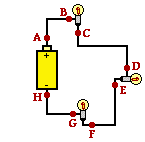53. Th diagram at the right shows three identical light bulbs wired in series. Several points along the circuit are labeled with letters. Compare the electric potential and the electric potential energy of the various points. For each comparison, use a greater than (>), less than (<), or approximately equal to (=) symbol.

 Electric Potential Comparison Potential Energy Comparison VA ____ VB PEA ____ PE B VB ____ VC PE B ____ PE C VC ____ VD PE C ____ PE D VD ____ VE PE D ____ PE E VE ____ VF PE E ____ PE F VF ____ VG PE F ____ PE G VG ____ VH PE G ____ PE H VH ____ VA PE H ____ PE A

54. Use proper schematic symbols to construct a diagram of a circuit powered by a 6-Volt battery that consists of two 3-ohm resistors connected in series. Place ammeters in series at a location such that the current through each resistor can be measured and in a location such that the overall current in the circuit can be measured. On the schematic diagram, use an unbroken arrow to indicate the direction of conventional current. Finally, indicate the ammeter readings on the diagram.

55. Use proper schematic symbols to construct a diagram of a circuit powered by a 6-Volt battery that consists of two 3-ohm resistors connected in parallel. Place an ammeter in series with each of the individual resistors in a manner that the current through each resistor can be measured. Place a third ammeter in a location such that the overall current in the circuit can be measured. On the schematic diagram, use an unbroken arrow to indicate the direction of conventional current. Finally, indicate the ammeter readings on the diagram.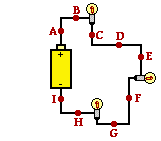56. Consider the diagram at the right of a series circuit. Each light bulb in the circuit has an identical resistance. Use the labeled points on the diagram to answer the following questions. Each question may have one, less than one, or more than one answer.

a. The electric potential at point A is the same as the electric potential at point(s) ____. Include all that apply, if any apply.

b. The electric potential at point C is the same as the electric potential at point(s) ____. Include all that apply, if any apply.

c. The electric potential at point F is the same as the electric potential at point(s) ____. Include all that apply, if any apply.

d. The electric potential at point I is the same as the electric potential at point(s) ____. Include all that apply, if any apply.

e. The electric potential difference between points A and B is the same as the electric potential difference between points ___ and ____. Include all that apply, if any apply.

f. The electric potential difference between points A and C is the same as the electric potential difference between points ___ and ____. Include all that apply, if any apply.

g. The electric potential difference between points A and F is the same as the electric potential difference between points ___ and ____. Include all that apply, if any apply.

h. The electric potential difference between points D and H is the same as the electric potential difference between points ___ and ____. Include all that apply, if any apply.

i. The current at point A is the same as the current at point(s) ___. Include all that apply, if any apply.

j. The current at point E is the same as the current at point(s) ___. Include all that apply, if any apply.

k. The current at point G is the same as the current at point(s) ___. Include all that apply, if any apply.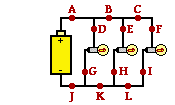57. Consider the diagram at the right of a parallel circuit. Each light bulb in the circuit has an identical resistance. Use the labeled points on the diagram to answer the following questions. Each question may have one, less than one, or more than one answer.

a. The electric potential at point A is the same as the electric potential at point(s) ____. Include all that apply, if any apply.

b. The electric potential at point D is the same as the electric potential at point(s) ____. Include all that apply, if any apply.

c. The electric potential at point J is the same as the electric potential at point(s) ____. Include all that apply, if any apply.

d. The electric potential difference between points A and J is the same as the electric potential difference between points ___ and ____. Include all that apply, if any apply.

e. The electric potential difference between points D and G is the same as the electric potential difference between points ___ and ____. Include all that apply, if any apply.

f. The current at point A is the same as the current at point(s) ___. Include all that apply, if any apply.

g. The current at point B is the same as the current at point(s) ___. Include all that apply, if any apply.

h. The current at point C is the same as the current at point(s) ___. Include all that apply, if any apply.

i. The current at point D is the same as the current at point(s) ___. Include all that apply, if any apply.

j. If the light bulb located between points D and G were to be replaced by a bulb of greater resistance, then the current at point(s) ___ would be decreased. Include all that apply, if any apply.

k. If the light bulb located between points D and G were to be replaced by a bulb of greater resistance, then the electric potential difference between points ___ and ___ would be increased. Include all that apply, if any apply.

l. If the light bulb located between points D and G were to go out, then the current would decrease at point(s) ___. Include all that apply, if any apply.

58. Consider the diagram below of a series circuit. For each resistor, use arrows to indicate the two locations where one would have to tap with the leads of a voltmeter in order to measure the voltage drop across the individual resistor. Finally, indicate the ammeter readings and the voltage readings.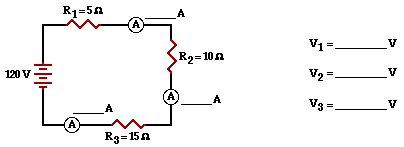59. Consider the diagram below of a parallel circuit. For each resistor, use arrows to indicate the two locations where one would have to tap with the leads of a voltmeter in order to measure the voltage drop across the individual resistor. Finally, indicate the ammeter readings and the voltage readings.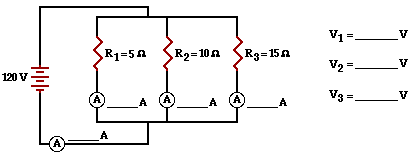### Part D: Qualitative Relationships Between Variables

60. A resistor with a resistance of R is connected to a battery with a voltage of V to produce a current of I. What would be the new current (in terms of I) if ...

a. ... the resistance is doubled and the same voltage is used?

b. ... the voltage is doubled and the same resistance is used?

c. ... the voltage is tripled and the resistance is doubled?

d. ... the voltage is doubled and the resistance is halved?

e. ... the voltage is halved and the resistance is doubled?

f. ... five times the voltage and one-third the resistance is used?

g. ... one-fifth the voltage and one-fourth the resistance is used?

61. A wire of length L and cross-sectional area A is used in a circuit. The overall resistance of the wire is R. What would be the new resistance (in terms of R) if ...

a. ... the length of the wire is doubled?

b. ... the cross-sectional area of the wire is doubled?

c. ... the length of the wire is doubled and the cross-sectional area of the wire is doubled?

d. ... the length of the wire is tripled and the cross-sectional area of the wire is doubled?

e. ... the length of the wire is halved and the cross-sectional area of the wire is tripled?

f. ... the length of the wire is tripled and the cross-sectional area of the wire is halved?

g. ... the length of the wire is tripled and the diameter of the wire is halved?

h. ... the length of the wire is tripled and the diameter of the wire is doubled?

62. An electric appliance with a current of I and a resistance of R converts energy to other forms at a rate of P when connected to a 120-Volt outlet. What would be the new power rating (in terms of P) if ...

a. ... the current is doubled (and the same 120-Volt outlet is used)?

b. ... the current is halved (and the same 120-Volt outlet is used)?

c. ... the resistance is doubled (and the same 120-Volt outlet is used)?

d. ... the resistance is halved (and the same 120-Volt outlet is used)?

e. ... the current is tripled (and the same 120-Volt outlet is used)?

f. ... the resistance is tripled (and the same 120-Volt outlet is used)?

63. An electric appliance with a current of I and a resistance of R is used for t hours during the course of a month. The cost of operating the appliance at 120-Volts is D dollars. What would be the new cost (in terms of D) if ...

a. ... the usage rate was doubled to 2t?

b. ... the usage rate was halved?

c. ... an appliance which drew twice the current (at 120 Volts) were used?

d. ... an appliance with twice the resistance (at 120 Volts) were used?

e. ... an appliance with one-half the resistance (at 120 Volts) were used?

f. ... the usage rate was doubled and an appliance with twice the resistance (at 120 Volts) were used?

g. ... the usage rate was halved and an appliance with twice the current (at 120 Volts) were used?

h. ... the usage rate was quartered and an appliance with twice the current (at 120 Volts) were used?64. Consider the diagram at the right of a parallel circuit. Each light bulb has an identical resistance of R and the battery voltage is V. Use the labeled points on the diagram to answer the following questions.

a. If the current at location A is I amperes, then the current at location B is ____ amperes. (Answer in terms of I.)

b. If the current at location A is I amperes, then the current at location D is ____ amperes. (Answer in terms of I.)

c. If the current at location A is I amperes, then the current at location L is ____ amperes. (Answer in terms of I.)

d. If the voltage of the battery is doubled, then the current at location A would be ____ (two times, four times, one-half, one-fourth, etc.) the original value.

e. If the voltage of the battery is doubled, then the current at location B would be ____ (two times, four times, one-half, one-fourth, etc.) the original value.

f. If the voltage of the battery is doubled, then the current at location D would be ____ (two times, four times, one-half, one-fourth, etc.) the original value.

g. Suppose that the resistance of the light bulb located between points D and G is doubled. This would result in the current measured at location G to ____ (increase, decrease, not be affected).

h. Suppose that the resistance of the light bulb located between points D and G is doubled. This would result in the electric potential difference between points D and G to ____ (increase, decrease, not be affected).

i. Suppose that the resistance of the light bulb located between points D and G is doubled. This would result in the current measured at location A to ____ (increase, decrease, not be affected).

j. Suppose that the resistance of the light bulb located between points D and G is doubled. This would result in the current measured at location E to ____ (increase, decrease, not be affected).

k. Suppose that the resistance of the light bulb located between points D and G is doubled. This would result in the current measured at location K to ____ (increase, decrease, not be affected).

### Part E: Problem-Solving and Circuit Analysis

65. If the current at a given point in a circuit is 2.5 Amps, then how many electrons pass that point on the circuit in a time period of 1 minute.

66. What is the resistance (in ohms) of a typical 40-Watt light bulb plugged into a 120-Volt outlet in your home?

67. Determine the length of nichrome wire (resistivity value = 150 x 10-8 ohm•m) required to produce a 1.00 mAmp current if a voltage of 1.5 Volts is impressed across it. The diameter of the wire is 1/16-th of an inch. (GIVEN: 2.54 cm = 1 inch)

68. Determine the total monthly cost of using the following appliances/household wires for the given amount of time if each is plugged into a 120-Volt household outlet. The cost of electricity is \$0.13 / kW•hr. (Assume that a month lasts for 30 days.)

 Appliance (with info from labels) Time (hours/day) Power (Watts) Energy Consumed Cost (\$) Hair Dryer (12 Amp) 0.10 Coffee Percolator (9.0 Amp) 0.10 Light Bulb (100 Watt) 8 Attic Fan (140 Watt) 10 Microwave Oven (8.3 Amps) 0.25

69. If the copper wire used to carry telegraph signals has a resistance of 10 ohms for every mile of wire, then what is the diameter of the wire. (Given: 1609 m = 1 mile. Resistivity of Cu = 1.7 x 10-8 ohm•m )

70. Determine the resistance of a 1500 Watt electric grill connected to a 120-Volt outlet.

71. Four resistors - 2-Ohms, 5-Ohms, 12-Ohms and 15-Ohms - are placed in series with a 12-Volt battery. Determine the current at and voltage drop across each resistor.

72. Four resistors - 2-Ohms, 5-Ohms, 12-Ohms and 15-Ohms - are placed in parallel with a 12-Volt battery. Determine the current at and voltage drop across each resistor.

#### Navigate to:

Review Session Home - Topic Listing

Electric Circuits - Home || Printable Version || Questions with Links

Answers to Questions:  All || #1-7 || #8-51 || #52-59 || #60-72

## You Might Also Like ...

Users of The Review Session are often looking for learning resources that provide them with practice and review opportunities that include built-in feedback and instruction. If that is what you're looking for, then you might also like the following:

The Calculator Pad includes physics word problems organized by topic. Each problem is accompanied by a pop-up answer and an audio file that explains the details of how to approach and solve the problem. It's a perfect resource for those wishing to improve their problem-solving skills.

2. Minds On Physics the App Series

Minds On Physics the App ("MOP the App") is a series of interactive questioning modules for the student that is serious about improving their conceptual understanding of physics. Each module of the series covers a different topic and is further broken down into sub-topics. A "MOP experience" will provide a learner with challenging questions, feedback, and question-specific help in the context of a game-like environment. It is available for phones, tablets, Chromebooks, and Macintosh computers. It's a perfect resource for those wishing to refine their conceptual reasoning abilities. Part 4 of the series includes topics in Electric Circuits.

Visit: MOP the App Home || MOP the App - Part 4